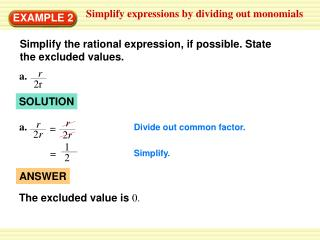# EXAMPLE 2 - PowerPoint PPT PresentationDownload PresentationEXAMPLE 2

Download Presentation## EXAMPLE 2

- - - - - - - - - - - - - - - - - - - - - - - - - - - E N D - - - - - - - - - - - - - - - - - - - - - - - - - - -
##### Presentation Transcript

1. r a. 2r 1 = 2 r r a. = 2r ANSWER 2r The excluded value is 0. Simplify expressions by dividing out monomials EXAMPLE 2 Simplify the rational expression, if possible. State the excluded values. SOLUTION Divide out common factor. Simplify.

2. 5x b. 5(x + 2) 5x 5 x b. = x = 5(x + 2) 5 (x + 2) (x + 2) ANSWER The excluded value is –2. Simplify expressions by dividing out monomials EXAMPLE 2 Simplify the rational expression, if possible. State the excluded values. SOLUTION Divide out common factor. Simplify.

3. 6m2 (m – 2) = m – 2 3 6 m2 6m3– 12m3 = c. 3 18m2 ANSWER The excluded value is0. 6m3– 12m3 c. 18m2 6m2 (m – 2) = 6 3 m2 Simplify expressions by dividing out monomials EXAMPLE 2 Simplify the rational expression, if possible. State the excluded values. SOLUTION Factor numerator and denominator. Divide out common factors. Simplify.

4. y d. 7 – y is already in simplest form. d. The expression ANSWER y The excluded value is7. 7 – y Simplify expressions by dividing out monomials EXAMPLE 2 Simplify the rational expression, if possible. State the excluded values. SOLUTION

5. 8x 7. 8. 6. 8x3 + 16x2 2s 1 3 x2 + 2x 2s2 + 8s 2c 2c 3s +12 4 a3 c + 5 c + 5 22a6 2 11a3 for Example 2 GUIDED PRACTICE 5. ANSWER The excluded value is0. The excluded value is– 5. ANSWER The excluded value is– 4. ANSWER The excluded values are 0 and– 2. ANSWER